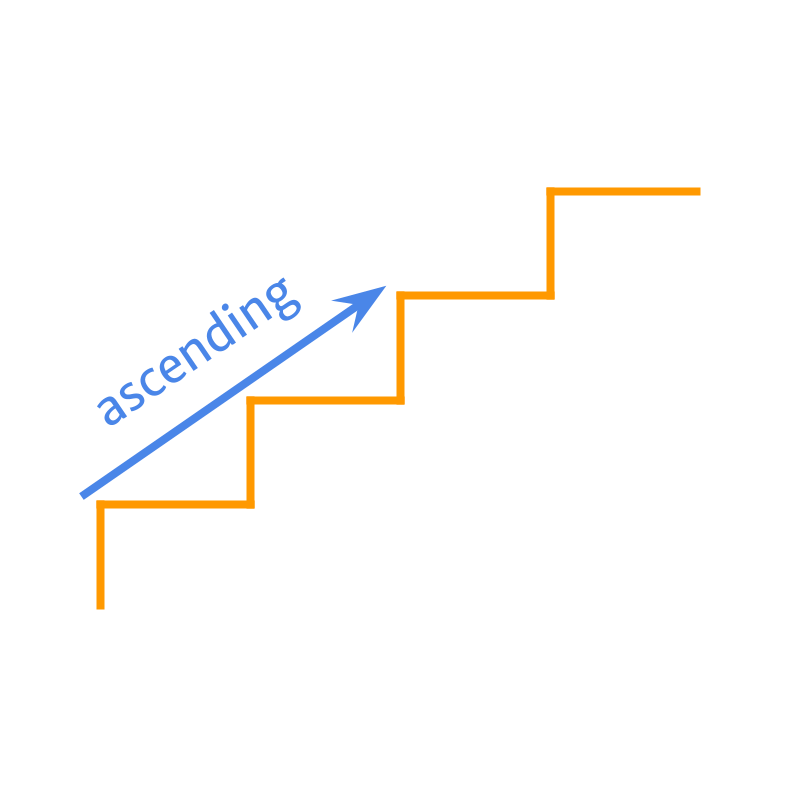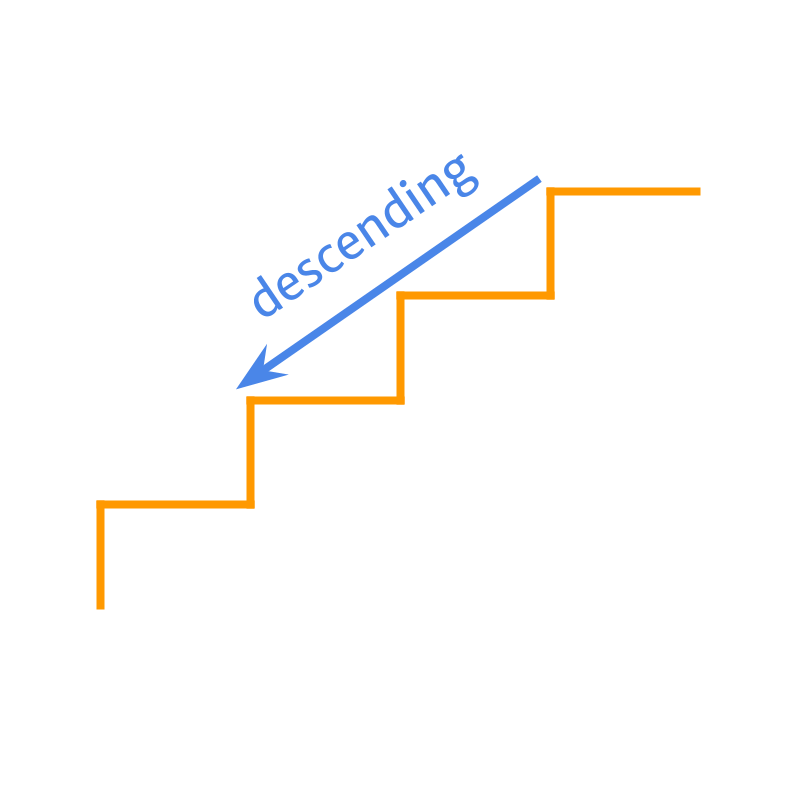maths > decimals

Comparing Decimals

what you'll learn...

overview

Comparison of Decimals :
Decimals are standardized fractions. Comparison based on place-value is applicable. The place value is extended to $1/{10}^{th}$$1 / {10}^{t h}$, $1/{100}^{th}$$1 / {100}^{t h}$, etc.

Decimals are directed numbers. Sign-property of comparison (as learned for integers) is applicable.

Comparison by place-value (as learned for whole numbers) is applicable with extended place-value order.
$\cdots <1/100<1/10<$$\cdots < 1 / 100 < 1 / 10 <$ units $<$$<$ tens $<$$<$ hundreds $<\cdots$$< \cdots$

Decimals can be compared to

•  find one as larger or smaller or equal.

•  arrange a few in ascending or descending order.

compare whole

Let us quickly review whole numbers arithmetics, integer arithmetics, and fractions arithmetics.

The number $7$$7$ is "larger" than the number $3$$3$.

The number $4$$4$ is "smaller" than the number $6$$6$.

The number $5$$5$ is "equal" to the number $5$$5$.

The number $53$$53$ is larger than $47$$47$.

Comparing the numbers $47$$47$ and $53$$53$.

By first principles : The quantities are matched. On comparing the quantities, $53$$53$ is found to be larger.

As a simplified procedure, the numbers in tens place value can be compared. $4$$4$ in $47$$47$ and $5$$5$ in $53$$53$. On comparing the tens place, $53$$53$ is found to be larger.

Note that once it is found that the tens place are not equal, the larger number is decided. The units place does not need to be compared. Because the tens place has $10$$10$ of units place".

This simplified procedure, "Comparison by Place-value" was introduced in whole numbers.

compare directed

$3$$3$ is larger than $-6$$- 6$, as \text{aligned:}3$\textrm{\left(a l i g \ne d\right\rangle} 3$ or $\text{received:}3$$\textrm{\left(r e c e i v e d\right\rangle} 3$ is larger than $\text{opposed:}6$$\textrm{\left(o p p o s e d\right\rangle} 6$ or $\text{given:}6$$\textrm{\left(g i v e n\right\rangle} 6$.

$-3$$- 3$ is larger than $-6$$- 6$, as $\text{opposed:}3$$\textrm{\left(o p p o s e d\right\rangle} 3$ or $\text{given:}3$$\textrm{\left(g i v e n\right\rangle} 3$ is larger than $\text{opposed:}6$$\textrm{\left(o p p o s e d\right\rangle} 6$ or $\text{given:}6$$\textrm{\left(g i v e n\right\rangle} 6$.

The comparison of directed numbers or integers is on the "aligned" or "received" or "positive" direction.

compare parts

The fraction $\frac{3}{4}$$\frac{3}{4}$ and $\frac{5}{8}$$\frac{5}{8}$are compared to find the larger one. But, considering the denominators (or place values) are different, the fractions cannot be compared without converting them to like fractions.

The fraction $\frac{3}{4}$$\frac{3}{4}$ is converted to $\frac{6}{8}$$\frac{6}{8}$ to make them like fractions.

Both $\frac{6}{8}$$\frac{6}{8}$ and $\frac{5}{8}$$\frac{5}{8}$ have the same place value $\frac{1}{8}$$\frac{1}{8}$ (ie: same denominator $8$$8$).

In this form, the numerators can be compared and $\frac{6}{8}$$\frac{6}{8}$ is larger, which means its equivalent fraction $\frac{3}{4}$$\frac{3}{4}$ is larger than $\frac{5}{8}$$\frac{5}{8}$.

recap

Given two whole numbers, integers, or fractions, they can be compared as

•  greater or larger

•  smaller or lesser

•  equal.

Comparison by First-principles : Two numbers representing count or quantities are matched. The amount that is more than other is larger, and the other number is smaller. If the amounts match then the numbers are equal.

Comparison by Place-value : For large number with units, tens, hundreds, ... digits, the comparison is started from the largest place value till one of the number is found to be larger or smaller.

Sign Property of comparison : To compare integers with positive and negative signs, the direction is also taken into consideration.

Comparison of Fractions :To compare fractions, they are converted to like fractions and the numerators are compared like an integer.

compare standardized fractions

Decimals are standardized fractions with place value of fractions as power of $10$$10$.

Given two decimals $0.7$$0.7$ and $0.6$$0.6$, the decimal $0.7$$0.7$ is larger than $0.6$$0.6$.

It is noted that
$0.7$$0.7$ means $7$$7$ counts of $1/{10}^{th}$$1 / {10}^{t h}$ parts and
$0.6$$0.6$ means $6$$6$ counts of $1/{10}^{th}$$1 / {10}^{t h}$ parts

So $7$$7$ counts or $0.7$$0.7$ is larger.

Given two decimals $0.7$$0.7$ and $0.62$$0.62$, the decimal $0.7$$0.7$ is larger than $0.62$$0.62$.

It is noted that
$0.7$$0.7$ means $7$$7$ counts of $1/{10}^{th}$$1 / {10}^{t h}$ parts and
$0.62$$0.62$ means $6$$6$ counts of $1/{10}^{th}$$1 / {10}^{t h}$ parts and $2$$2$ counts of $1/{100}^{th}$$1 / {100}^{t h}$ parts.

So $7$$7$ counts or $0.7$$0.7$ is larger.

Given two decimals $0.7$$0.7$ and $1.2$$1.2$, the decimal$1.2$$1.2$ is larger than $0.7$$0.7$.

It is noted that
$0.7$$0.7$ is $7$$7$ counts of $1/{10}^{th}$$1 / {10}^{t h}$ parts and
$1.2$$1.2$ is $1$$1$ whole and $2$$2$ counts of $1/10\left(th\right)$$1 / 10 \left(t h\right)$ parts.

So $1$$1$ count of whole or $1.2$$1.2$ is larger.

compare directed decimals

Given two decimals $-0.7$$- 0.7$ and $0.62$$0.62$, the decimal $0.62$$0.62$ is larger than $-0.7$$- 0.7$.

It is noted that
$-0.7$$- 0.7$ represents $\text{opposed:}7$$\textrm{\left(o p p o s e d\right\rangle} 7$ counts of $1/{10}^{th}$$1 / {10}^{t h}$ parts and
$0.62$$0.62$ represents \text{aligned:}6$\textrm{\left(a l i g \ne d\right\rangle} 6$ counts of $1/{10}^{th}$$1 / {10}^{t h}$ parts and \text{aligned:}2$\textrm{\left(a l i g \ne d\right\rangle} 2$ counts of $1/100\left(th\right)$$1 / 100 \left(t h\right)$ parts.

So, the positive value $0.62$$0.62$ is larger.

Given two decimals $-0.5$$- 0.5$ and $-0.2$$- 0.2$, the decimal $-0.2$$- 0.2$ is larger than $-0.5$$- 0.5$.

It is noted that
$-0.5$$- 0.5$ means $\text{opposed:}5$$\textrm{\left(o p p o s e d\right\rangle} 5$ counts of $1/{10}^{th}$$1 / {10}^{t h}$ parts and
$-0.2$$- 0.2$ means $\text{opposed:}2$$\textrm{\left(o p p o s e d\right\rangle} 2$ counts of $1/{10}^{th}$$1 / {10}^{t h}$ parts.

So, negative of smaller absolute value $-0.2$$- 0.2$ is larger.

place value order

In whole numbers, we learned that the place-value order is
units $<$$<$ tens $<$$<$ hundreds $<\cdots$$< \cdots$.

In decimals, the place-value is extended with $\frac{1}{10}$$\frac{1}{10}$, $\frac{1}{100}$$\frac{1}{100}$, etc.

The revised place-value order for decimals is "$\cdots <1/100<1/10<$$\cdots < 1 / 100 < 1 / 10 <$ units $<$$<$ tens $<$$<$ hundreds $<\cdots$$< \cdots$".

The place-value order is extended for decimals as in
$\cdots <1/100<1/10<$$\cdots < 1 / 100 < 1 / 10 <$ units $<$$<$ tens $<$$<$ hundreds $<\cdots$$< \cdots$

summary of decimal comparison

Comparison of Decimals :
Decimals are standardized fractions of whole numbers. Comparison based on place-value is applicable. The place value is extended to $1/{10}^{th}$$1 / {10}^{t h}$, $1/{100}^{th}$$1 / {100}^{t h}$, etc.

Decimals are directed numbers. Sign-property of comparison (as learned for integers) is applicable.
•  When comparing +ve and +ve, the number with larger value is larger than the other.
•  When comparing +ve and -ve, the +ve number is larger irrespective of the absolute values of the numbers.
•  When comparing -ve and -ve, the number with smaller absolute value is larger than the other.

Comparison by place-value (as learned for whole numbers) is applicable with extended place-value order.
$\cdots <1/100<1/10<$$\cdots < 1 / 100 < 1 / 10 <$ units $<$$<$ tens $<$$<$ hundreds $<\cdots$$< \cdots$

examples

Which of the following is larger than the other? $-3.41$$- 3.41$ or $-3.41$$- 3.41$
The answer is "they are equal"

Which of the following step help to find the larger among $-.23$$- .23$ and $-1.56$$- 1.56$?
The answer is "compare the absolute values $.23$$.23$ and $1.56$$1.56$". The smaller absolute value is the larger number.

Which of the following is smaller than the other? $-21.83$$- 21.83$ or $-2.661$$- 2.661$
The answer is "$-21.83$$- 21.83$".

Find the smaller number among $-2.3$$- 2.3$ and $-.0099$$- .0099$
The answer is "$-2.3$$- 2.3$".

up or down

We have learned about comparing decimals. This can be used to order more than two decimals in ascending or descending orders. Let us quickly review these.The word 'ascend' means: to go up or climb.The word 'descend' means: to move down.

examples

Arrange the numbers in ascending order $-23.4$$- 23.4$, $7.3$$7.3$, $42.3$$42.3$.
The answer is "$-23.4$$- 23.4$, $7.3$$7.3$, $42.3$$42.3$"

Arrange the numbers in descending order $8.73$$8.73$, $.9$$.9$, $-9.9$$- 9.9$.
The answer is "$8.73$$8.73$, $.9$$.9$, $-9.9$$- 9.9$"

Arrange the numbers in ascending order $-8$$- 8$, $-8.43$$- 8.43$, $-8.4$$- 8.4$.
The answer is "$-8.43$$- 8.43$, $-8.4$$- 8.4$, $-8$$- 8$".

Arrange the numbers in descending order $0.002$$0.002$, $0.02$$0.02$, $0.0002$$0.0002$.
The answer is "$0.02$$0.02$, $0.002$$0.002$, $0.0002$$0.0002$"

Arrange the numbers in descending order $0.5$$0.5$, $-5$$- 5$, $5$$5$, $-0.5$$- 0.5$.
The answer is "$5$$5$, $.5$$.5$, $-.5$$- .5$, $-5$$- 5$"

Arrange the numbers in ascending order $-2.3$$- 2.3$, $0$$0$, $4.2$$4.2$.
The answer is "$-2.3,0,4.2$$- 2.3 , 0 , 4.2$"

summary

Comparison of Decimals :
Decimals are standardized fractions of whole numbers. Comparison based on place-value is applicable. The place value is extended to $1/{10}^{th}$$1 / {10}^{t h}$, $1/{100}^{th}$$1 / {100}^{t h}$, etc.

Decimals are directed numbers. Sign-property of comparison (as learned for integers) is applicable.

•  When comparing +ve and +ve, the number with larger value is larger than the other.

•  When comparing +ve and -ve, the +ve number is larger irrespective of the absolute values of the numbers.

•  When comparing -ve and -ve, the number with smaller absolute value is larger than the other.

Comparison by place-value (as learned for whole numbers) is applicable with extended place-value order.
$\cdots <1/100<1/10<$$\cdots < 1 / 100 < 1 / 10 <$ units $<$$<$ tens $<$$<$ hundreds $<\cdots$$< \cdots$

Decimals can be compared to

•  find one as larger or smaller or equal.

•  arrange a few in ascending or descending order.

Outline